VOPlot – The VOTable plotting utility (Version 1.0)Introduction

## Introduction

The standalone version of the VOPlot (VOTable Plot) is an application for plotting different astronomical graphs using data stored in VOTable format. Its web-based version is integrated with the VizieR Catalogue Service and can be used to plot any catalogue by selecting output layout as "Plot (VOPlot)". Click here for Release Notes and disclaimer information.

VOPlot has been developed as a part of the Virtual Observatory - India initiative by Persistent Systems and the Inter-University Centre for Astronomy and Astrophysics (IUCAA), in collaboration with Centre de Données astronomiques de Strasbourg (CDS), with a support from the European AVO project. The collaboration between VO-I and CDS extends to several related projects.The VO-I project is supported by the Ministry of Information and Communication Technology of the Governement of India.

VOPlot uses Ptplot 5.2, a 2D data plotter and histogram tool implemented in Java. Ptplot has been developed at EECS department at the University of California, Berkeley.

## Getting Started

To use the standalone version of VOPlot, you will need to download the executable jar file named voplot.jar. The file can be executed by typing the following command at the command prompt:

java -jar voplot.jar

This will open a java application window as shown in the Fig. 0. To use data from a VOTable you will need to load it first. You can either specify the URL of a VOTable or specify the path of a VOTable on your machine by using the “Browse” button. The name of the selected VOTable will appear in the text box. Click on the “Load” button to start using the VOTable. The message on the status bar will indicate whether the file was loaded successfully.

#### Figure 0Top

## Plotting Scatter Plots

To draw a plot of one columns against the other,

1. Select the column to be plotted on the X-axis.
2. Select the column to be plotted on the Y-axis.
3. Click "Plot" button.

You can see the scatter plot. If required, you can plot data points on a log scale by setting the option appropriately using the "Log" checkbox.

#### Figure 1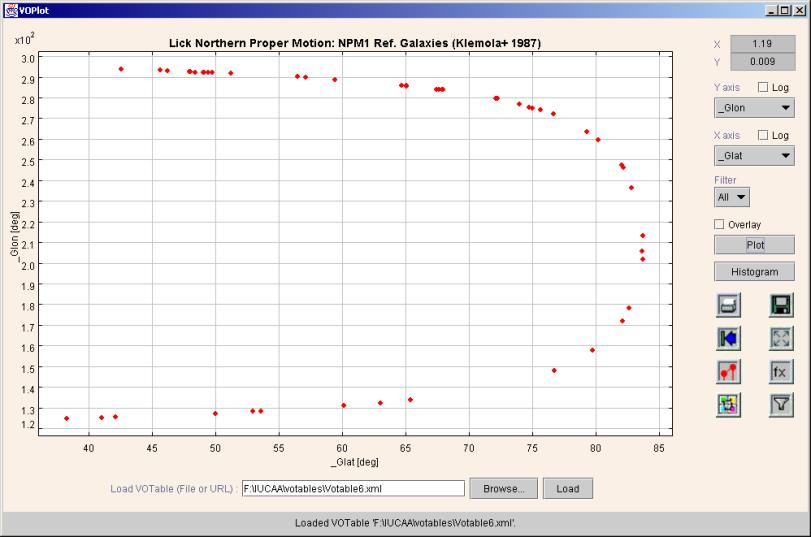You can draw connected line plots by checking the “Connected” checkbox in the Plot Properties dialog box.

To change various plot properties, such as title, labels ranges etc., click on the format icon.

Figure 2Top

## Plotting Histograms

To draw the histogram of a column

1. Select the column on X-axis.
2. Click "Histogram" button.

A sample histogram is shown in Figure 3.

Figure 3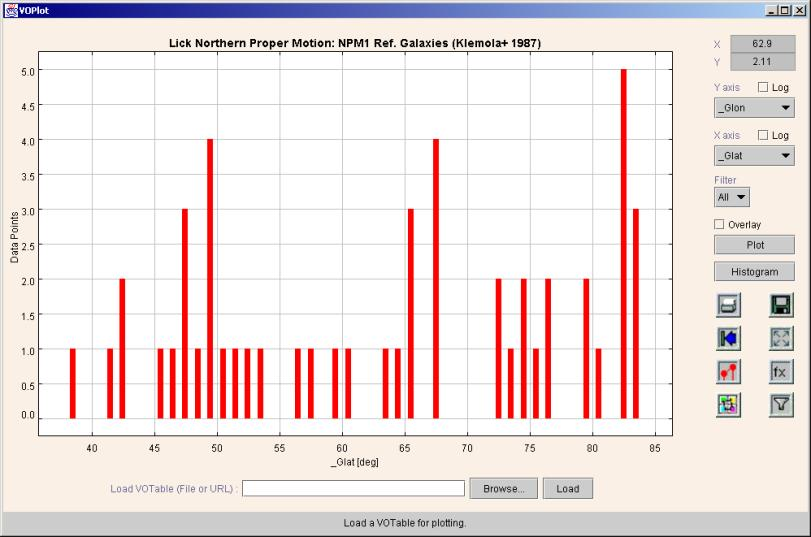To plot the histogram bar heights on a logarithmic scale, check the "Log" checkbox corresponding to the Y-axis If you want to plot the histogram of data points on a log scale, then check the "Log" checkbox corresponding to the X-axis.

When drawing histograms on a logarithmic Y-axis, the bars with height 1 cannot be drawn. You can force the VOPlot to draw such bars by incrementing the height of all the bars by 1. This is done by checking the “Incremented Y” checkbox in the Histogram Properties dialog box.

To change the histogram properties, including the bin width, click on the format icon.

Figure 4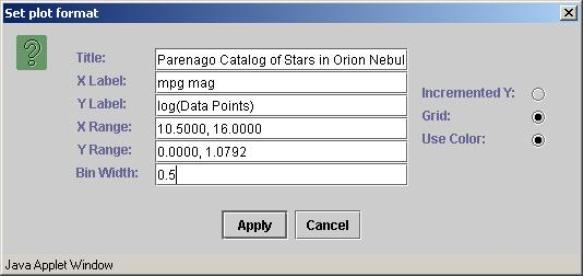Top

## Zooming

To zoom in, drag the left mouse button down and to the right to draw a box around an area that you want to see in detail.

To zoom out, drag the left mouse button up and to the left.

To get the original graph click on the "Reset" icon represented by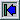.

Top

## Overlaying Plots

To overlay plots (simultaneously viewing multiple plots with similar range on the same axes system)

1. Select column to be plotted on the X-axis.
2. Select column to be plotted on the Y-axis.
3. Click "Plot" button.
4. Select column to be plotter on X-axis (represents another plot).
5. Select column to be plotter on Y-axis (represents another plot)
6. Set the "Overlay" option.
7. Click "Plot" button.

You can see the second plot overlaid on the first one if the second one is in the same range as the previous one. Only that portion of the second plot is visible that is within the already plotted range.

An example of overlaid plots is shown in Figure 5.

Figure 5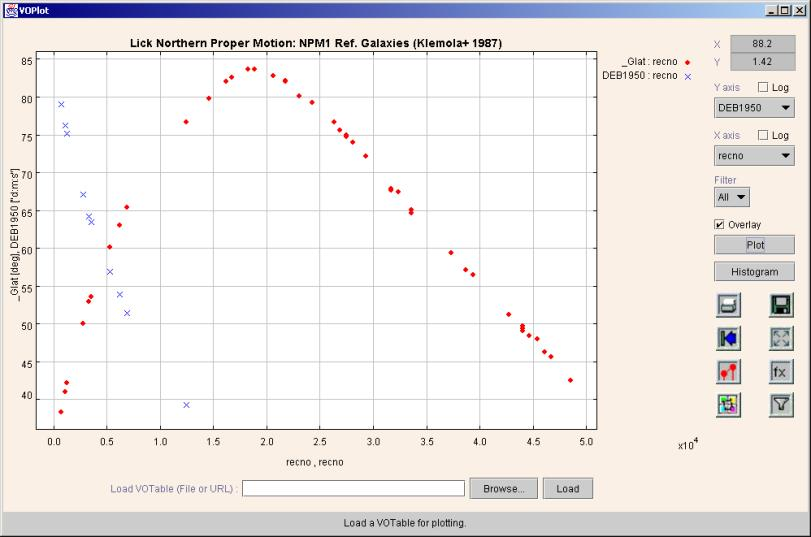To see the plots overlaid together (even if they don’t lie in the same range) click on the fill icon represented by. Figure 6 shows the complete datasets of all the overlaid plots in the graph above. A different marker will be used for each plot, to allow one to differentiate between the plots.

Figure 6Top

## Overlaying Histograms

To overlay histograms (simultaneously viewing multiple histograms with similar range)

1. Select column to be plotted on the X-axis.
2. Click "Histogram" button.
3. Select column to be plotter on X-axis (represents another plot)
4. Set the "Overlay" option.
5. Click "Histogram" button.

The second histogram is seen only if it lies within the same X and Y range as the first one. Click on the fill icon represented byto see both histograms irrespective of the range.  A different color will be used for each histogram, to allow one to differentiate between the histograms.

Example of overlaid histogram is shown in Figure 7.

Figure 7Top

## Transformations on columns

One can create new columns by defining transformations on them. You can use expressions with arithmetic operators, trigonometric functions, and miscellaneous functions shown below to create new columns. You can use transformed columns for plotting.

### Operators Allowed

 + Addition - Subtraction * Multiplication / Division

### Functions

 log(a) Log to the base 10. ln(a) Log to the base e. pow(a,b) "a" raised to power of "b". sqrt(a) Square root of "a". exp(a) Euler's number e raised to the power of "a". dexp(a) 10 raised to the power of "a". cos(a) Trigonometric cosine of an angle. a = an angle in radians. acos(a) Arc cosine of an angle sin(a) Trigonometric sine of an angle. a = an angle in radians. asin(a) Arc sine of an angle tan(a) Trigonometric tangent of an angle. a = an angle in radians. atan(a) Arc tangent of an angle toradians(a) Converts an angle measured in degrees to an equivalent angle measured in radians. todegrees(a) Converts an angle measured in radians to an equivalent angle measured in degrees.

##### Creating new columns
1. To define transformations on columns click on the Transformations Icon represented byto display the Transformations Dialog Box.
2. In the Transformations Dialog Box, you can see the column names, their Id (used for expression building) and the expression with which they were created.
3. For creating a new column, type in the new column name (example f1Glat) and the expression using the functions shown above. Example: \$1+sin(\$3)*4

The dialog box for creating new columns is shown in Figure 8.

Figure 8Top

## Creating data subsets by applying filters

You can create new data subsets by defining filters on them. You can use filter definition with relational operators and logical operators with operators and functions shown above to create new data subsets. Data subsets can be used for plotting.

##### Operators Allowed

 < Less than <= Less than or equal to > Greater than >= Greater than or equal to == Equal to != Not equal to && And || Or ! Not

##### Creating new data subsets
1. To define new data subsets on columns click on the Filters Icon represented byto display the "Create new data subsets" dialog box.
2. In this dialog box, you can see the column names, their Id (used for expression building) and the expression with which they were created. You can also see the data subsets that you previously created with their names and conditions.
3. For creating a new data subset, type in the new subset name (example f1Glat) and the filter definition using the functions and relational operators shown in table above. You can also make use for arithmetic operators and functions listed for "Transformations on columns". Example: \$1 > 0 || (\$3! = 0 && \$2 > 100)

The dialog box for creating data subsets is shown in Figure 9.

Figure 9Once a data subset is created you can plot data from the subset. This can be done by choosing the data subset from the Filters combo box in the main applet window.

Note: "All" represents the complete data. Only data points satisfying the filter condition will be considered for plotting.

Top

## Statistical Functions on Plotted Data

Statistical functions can be applied on the plotted data.

##### Applying Statistical Functions
1. To apply statistical functions, click on "Statistics for the plot" icon represented by. The "Plot Statistics" dialog box will open.
2. You can choose the data subset from the filter combo box. Note: "All" represents the complete data.
3. Select X, Y and Z axes and click "Calculate" button. Note: Default columns are picked up from the current plot. The results are displayed on the same window.

The dialog box is divided into two tabs – basic and advanced functions. The basic functions require only one data array, while the advanced functions require two or three data arrays as parameters.

The following statistical functions are currently supported.

 Sr. No. Function name Input Columns 1 Number of observations X 2 Range X 3 Minimum X 4 Maximum X 5 Mean X 6 Variance X 7 Standard deviation X 8 Skew X 9 Kurtosis X 10 Linear correlation X, Y 11 Significance (t) for Linear correlation X, Y 12 Probability for Linear correlation X, Y 13 Rank correlation X, Y 14 Partial correlation X, Y, Z

VOPlot, by default, takes the complete dataset into consideration while evaluating the statistical functions. However you can force it to consider only the plotted data range by selecting the "Use only plot data" checkbox. This will evaluate the statistical functions for the currently plotted columns, along with the applied filter, if any, in the current axes ranges.

Sample dialog box with basic tab selected showing plot statistics is shown in Figure 10.

Figure 10Sample "Advanced Functions" tab is shown in Figure 11.

Figure 11Top

## Saving plot as an image

For saving the plot as an image, plot the desired image and click on save icon represented by. The save as dialog box will appear. Select a file and click on OK to save the image as an EPS file onto your machine. Currently, the image can only be saved as an EPS file